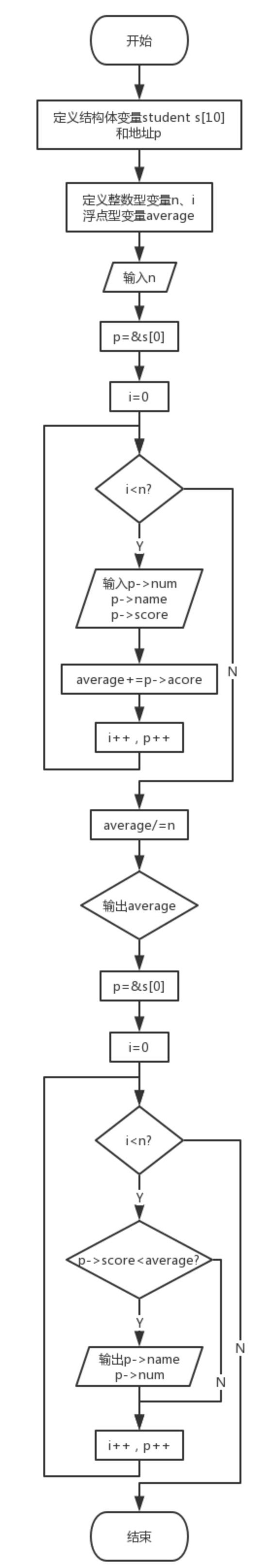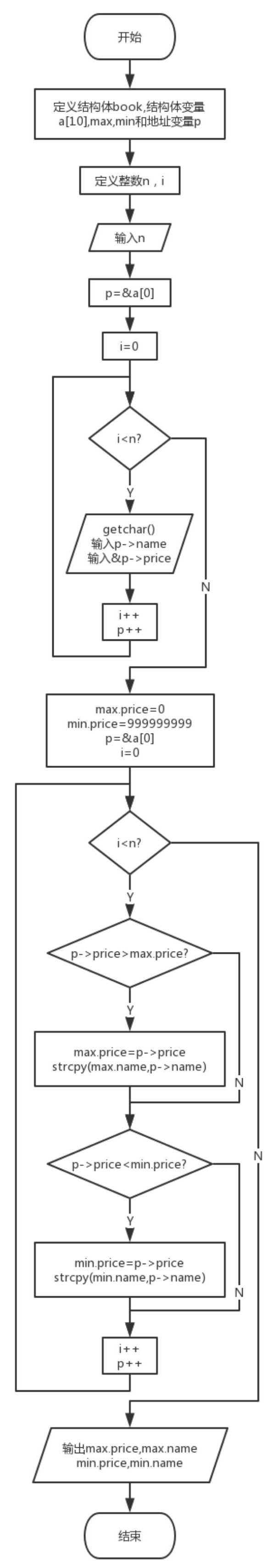# 2018C语言第三次作业

• 要求一

2.struct sk{int a; char *str)}*p;   p->str++ 中的++ 加向？

++加向srt的地址。

• 要求二

1.设计思路

(1)主要描述题目算法

(2)流程图2.实验代码

#include<stdio.h>
#include<stdlib.h>
#include<string.h>
struct student
{
char num;
char name;
int score;
}s,*p;
int main()
{
int n,i;
double average=0;
scanf("%d",&n);
p=&s;
for(i=0;i<n;i++,p++)
{
scanf("%s%s%d",p->num,p->name,&p->score);
average+=p->score;
}
average/=n;
printf("%.2lf",average);
p=&s;
for(i=0;i<n;i++,p++)
{
if(p->score<average)
{
printf("\n%s %s",p->name,p->num);
}
}
return 0;
}


3.本题调试过程碰到问题及解决办法

本题课堂进行了类似的练习所以没有错误，需要注意的应该就是输出格式，这次编程中使用了不同于课堂练习的地址变量指向结构体成员的方式。1.设计思路

(1)主要描述题目算法

(2)流程图2.实验代码

#include<stdio.h>
#include<stdlib.h>
#include<string.h>
struct book
{
char name;
double price;
}a,*p,max,min;
int main()
{
int n,i;
scanf("%d",&n);
p=&a;
for(i=0;i<n;i++,p++)
{
getchar();
gets(p->name);
scanf("%lf",&p->price);
}
max.price=0;
min.price=999999999;
p=&a;
for(i=0;i<n;i++,p++)
{
if(p->price>max.price)
{
max.price=p->price;
strcpy(max.name,p->name);
}
if(p->price<min.price)
{
min.price=p->price;
strcpy(min.name,p->name);
}
}
printf("%.2lf, %s\n%.2lf, %s",max.price,max.name,min.price,min.name);
return 0;
}

3.本题调试过程碰到问题及解决办法1.设计思路

(1)主要描述题目算法

(2)流程图2.实验代码

int set_grade(struct student *p,int n)
{
int count=0;
while(n--)
{
if(p->score<=100&&p->score>=85)
else if(p->score>=70)
else if(p->score>=60)
else
{
count++;
}
p++;
}
return count;
}


3.本题调试过程碰到问题及解决办法• 要求三

1.指针与结构作业中题目所使用的知识点

①结构体的声名方式，及其内部成员的定义；

②结构体的指针变量应用和函数应用。posted @ 2018-04-23 23:26  C6H6  阅读(196)  评论(0编辑  收藏  举报